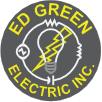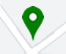Toll Free: (877) 385-8624

# Electrical Formulas

 To Find Direct Current Alternating Current Single Phase Two-Phase* Four-Wire Three Phase Amperes when Horsepower is known HP x 746 E x EFF HP x 746 E x EFF x PF x 2 HP x 746 2 x E x EFF x PF HP x 746 E x EFF x PF x 1.73 Amperes when Kilowatts are known KW x 1000 E KW x 1000 E x PF KW x 1000 2 x E x PF KW x 1000 E x PF x 1.73 Amperes when "KVA" is known KVA x 1000 E KVA x 1000 KVA x 1000 E x 1.73 Kilowatts E x I 1000 E x I x PF 1000 I x E x 2 x PF 1000 E x I x 1.73 x PF 1000 Kilovolt-Amperes "KVA" - I x E 1000 I x E x 2 1000 E x I x 1.73 1000 Horsepower (Output) E x I x EFF 746 E x I x EFF x PF 746 I x E x 2 x EFF x PF 746 E x I x EFF x PF x 1.73 746

E = Voltage
I = Amps
PF = Power Factor
EFF = Efficiency
HP = HorsepowerSingle phase formulas do not use (2 or 1.73)

Note: Direct current formulas do not use (PF, 2, or 1.73)

• Two phase-four wire formulas do not use (1.73)
• Three phase formulas do not use (2)
• * For three-wire, two phase circuits the current in the common conductor is 1.41 times that in either of the other two conductors.
Toll Free: (877-385-8624, Ph: (740) 385-8624 Fx: (740) 385-0024

### 8/30/21 We are in the process of upgrading servers. Email service will be disrupted during the cutover. Please use edgreenelectric@gmail.com or call or fax numbers below.

##### Corporate Office:

406 West 2nd Street
Logan, Ohio 43138Hours: 8:00 AM to 5:00 PM M-F